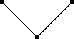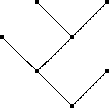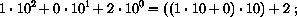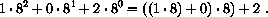# Section 31Designing Accumulator-Style Functions

Section 30 illustrated with two examples the need for accumulating extra knowledge. In some cases, the accumulation makes it easier to understand a function; in others it is necessary for the function to work properly. In both cases, however, we first chose one of the available design recipes, inspected the function, and then revised or fixed it. Put more generally, adding an ACCUMULATOR, that is, a parameter that accumulates knowledge, is something that we add to a function after we have designed a function, not before.

The keys to the development of an accumulator-style function are:

1. to recognize that the function benefits from, or needs, an accumulator;

2. to understand what the accumulator represents.

The first two subsections address these two questions. Because the second one is a difficult topic, the third subsection illustrates how to formulate precise claims about accumulators. More concretely, in this section, we transform several standard recursive functions into functions that use auxiliary functions with accumulators.

## 31.1  Recognizing the Need for an Accumulator

Recognizing the need for accumulators is not an easy task. We have seen two reasons, and they are the most prevalent reasons for adding accumulator parameters. In either case, it is critical that we first built a complete function based on a design recipe. Then we study the function and look for one of the following characteristics:

1. If the function is structurally recursive and if the result of a recursive application is processed by an auxiliary, recursive function, then we should consider the use of an accumulator parameter.

Take the function `invert` as an example:

```;; `invert : (listof X)  ->  (listof X)`
;; to construct the reverse of `alox`
;; structural recursion
(define (invert alox)
(cond
[(empty? alox) empty]
[else (make-last-item (first alox) (invert (rest alox)))]))

;; `make-last-item : X (listof X)  ->  (listof X)`
;; to add `an-x` to the end of `alox`
;; structural recursion
(define (make-last-item an-x alox)
(cond
[(empty? alox) (list an-x)]
[else (cons (first alox) (make-last-item an-x (rest alox)))]))
```

The result of the recursive application produces the reverse of the rest of the list. It is processed by `make-last-item`, which adds the first item to the reverse of the rest and thus creates the reverse of the entire list. This second, auxiliary function is also recursive. We have thus identified a potential candidate. It is now time to study some hand-evaluations, as we did in section 30.1, to see whether an accumulator helps.

2. If we are dealing with a function based on generative recursion, we are faced with a much more difficult task. Our goal must be to understand whether the algorithm can fail to produce a result for inputs for which we expect a result. If so, adding a parameter that accumulates knowledge may help. Because these situations are complex, we postpone the discussion of an example until section 32.2.

These two situations are by no means the only ones; they are just the most common ones. To sharpen our perception, we will discuss an additional array of possibilities in the following section.

## 31.2  Accumulator-Style Functions

When we have decided that an accumulator-style function is necessary, we introduce it in two steps:

Setting-up an accumulator:
First, we must understand what knowledge the accumulator needs to remember about the parameters proper and then how it is to remember it. For example, for the conversion of relative distances to absolute distances, it suffices to accumulate the total distance encountered so far. For the routing problem, we needed the accumulator to remember every node inspected so far. Thus the first accumulator was just a number, the second one a list of nodes.

The best way to discuss the accumulation process is to introduce a template of the accumulator-style function via a `local` definition and to name the parameters of the function proper differently from those of the auxiliary function.

Let's take a look at the `invert` example:

```;; `invert : (listof X)  ->  (listof X)`
;; to construct the reverse of `alox`
(define (invert alox0)
(local (;; `accumulator` ...
(define (rev alox accumulator)
(cond
[(empty? alox) ...]
[else
... (rev (rest alox) ... ( first alox) ... accumulator)
... ])))
(rev alox0 ...)))
```

Here we have a definition of `invert` with an auxiliary function `rev` in template form. This auxiliary template has one parameter in addition to those of `invert`: the accumulating parameter. The box in the recursive application indicates that we need an expression that maintains the accumulation process and that this process depends on the current value of `accumulator` and `(first alox)`, the value `rev` is about to forget.

Clearly, `invert` cannot forget anything, because it only reverses the order of items on the list. Hence we might just wish to accumulate all items that `rev` encounters. This means

1. that `accumulator` stands for a list, and

2. that it stands for all those items in `alox0` that precede the `alox` argument of `rev`.

For the second part of the analysis, it is critical that we can distinguish the original argument, `alox0`, from the current one, `alox`.

Now that we know the rough purpose of the accumulator, let's consider what the first value should be and what we should do for the recursion. When we apply `rev` in the body of the local-expression, it receives `alox0`, which means that it hasn't encountered any of its items. The initial value for `accumulator` is `empty`. When `rev` recurs, it has just encountered one extra item: `(first alox)`. To remember it, we can `cons` it onto the current value of accumulator.

Here is the enhanced definition:

```;; `invert : (listof X)  ->  (listof X)`
;; to construct the reverse of `alox`
(define (invert alox0)
(local (;; `accumulator` is the reversed list of all those items
;; on `alox0` that precede `alox`
(define (rev alox accumulator)
(cond
[(empty? alox) ...]
[else
... (rev (rest alox) (cons (first alox) accumulator))
...])))
(rev alox0 empty)))
```

A close inspection reveals that `accumulator` is not just the items on `alox0` that precede but a list of these items in reverse order.

Exploiting an accumulator:
Once we have decided what knowledge the accumulator maintains and how it is maintained, we can move to the question of how to exploit this knowledge for our function.

In the case of `invert`, the answer is almost obvious. If `accumulator` is the list of all items on `alox0` that precede `alox` in reverse order, then, if `alox` is empty, `accumulator` stands for the reverse of `alox0`. Put differently: if `alox` is `empty`, `rev`'s answer is `accumulator`, and that is the answer we want in both cases:

```;; `invert : (listof X)  ->  (listof X)`
;; to construct the reverse of `alox`
(define (invert alox0)
(local (;; `accumulator` is the reversed list of all those items
;; on `alox0` that precede `alox`
(define (rev alox accumulator)
(cond
[(empty? alox) accumulator]
[else
(rev (rest alox) (cons (first alox) accumulator))])))
(rev alox0 empty)))
```

The key step of this development is the precise description of the role of `accumulator`. In general, an ACCUMULATOR INVARIANT describes a relationship between the argument proper of the function, the current argument of the auxiliary function, and the accumulator that must always hold when an accumulator-style function is used.

## 31.3  Transforming Functions into Accumulator-Style

The most complex part of the design recipe is the requirement to formulate an accumulator invariant. Without that we cannot produce functioning accumulator-style functions. Because formulating invariants is clearly an art that deserves a lot of practice, we practice it in this section with three small, well-understood structural functions that do not need an accumulator. The section concludes with a group of exercises concerning this step.

For the first example, consider the function `sum`:

```;; `sum : (listof number)  ->  number`
;; to compute the sum of the numbers on `alon`
;; structural recursion
(define (sum alon)
(cond
[(empty? alon) 0]
[else (+ (first alon) (sum (rest alon)))]))
```

Here is the first step toward an accumulator version:

```;; `sum : (listof number)  ->  number`
;; to compute the sum of the numbers on `alon0`
(define (sum alon0)
(local (;; `accumulator` ...
(define (sum-a alon accumulator)
(cond
[(empty? alon) ...]
[else
... (sum-a (rest alon) ... ( first alon) ... accumulator)
... ])))
(sum-a alon0 ...)))
```

As suggested by our first step, we have put the template for `sum-a` into a `local` definition, added an accumulator parameter, and renamed `sum`'s parameter.

Our goal is to develop an accumulator invariant for `sum`. To do so, we must consider how `sum` proceeds and what the goal of the process is. Like `rev`, `sum-a` processes the numbers on the list one by one. The goal is to add up these numbers. This suggests that `accumulator` represents the sum of the numbers seen so far:

```...
(local (;; `accumulator` is the sum of the numbers that preceded
;; those in `alon` on `alon0`
(define (sum-a alon accumulator)
(cond
[(empty? alon) ...]
[else
... (sum-a (rest alon) (+ (first alon) accumulator))
... ])))
(sum-a alon0 0)))
```

When we apply `sum-a` we must use `0` as the value of `accumulator`, because it hasn't processed any of the numbers on `alon` yet. For the second clause, we must add `(first alon)` to `accumulator` so that the invariant holds again for the function application.

Given a precise invariant, the rest is straightforward again. If `alon` is `empty`, `sum-a` returns `accumulator` because it represents the sum of all numbers on `alon` now. Figure 88 contains the final definition of the accumulator-style version of `sum`.

 ```;; `sum : (listof number)  ->  number` ;; to compute the sum of the numbers on `alon0` (define (sum alon0) (local (;; `accumulator` is the sum of the numbers that preceded ;; those in `alon` on `alon0` (define (sum-a alon accumulator) (cond [(empty? alon) accumulator] [else (sum-a (rest alon) (+ (first alon) accumulator))]))) (sum-a alon0 0))) ``` ```;; `! : N  ->  N` ;; to compute n · (n - 1) · ... · 2 · 1 (define (! n0) (local (;; `accumulator` is the product of all natural numbers in [`n0`, `n`) (define (!-a n accumulator) (cond [(zero? n) accumulator] [else (!-a (sub1 n) (* n accumulator))]))) (!-a n0 1))) ``` Figure 88:  Some simple accumulator-style functions

Let's compare how the original definition of `sum` and the accumulator-style definition produce an answer for the same input:

 ``` (sum (list 10.23 4.50 5.27)) = (+ 10.23 (sum (list 4.50 5.27))) = (+ 10.23 (+ 4.50 (sum (list 5.27)))) = (+ 10.23 (+ 4.50 (+ 5.27 (sum empty)))) = (+ 10.23 (+ 4.50 (+ 5.27 0))) = (+ 10.23 (+ 4.50 5.27)) = (+ 10.23 9.77) = 20.0 ``` ``` (sum (list 10.23 4.50 5.27)) = (sum-a (list 10.23 4.50 5.27) 0) = (sum-a (list 4.50 5.27) 10.23) = (sum-a (list 5.27) 14.73) = (sum-a empty 20.0) = 20.0 ```
On the left side, we see how the plain recursive function descends the list of numbers all the way to the end and sets up addition operations on the way. On the right side, we see how the accumulator-style version adds up the numbers as it goes. Furthermore, we see that for each application of `sum-a` the invariant holds with respect to the application of `sum`. When `sum-a` is finally applied to `empty`, the accumulator is the final result, and `sum-a` returns it.

Exercise 31.3.1.   A second difference between the two functions concerns the order of addition. While the original version of `sum` adds up the numbers from right to left, the accumulator-style version adds them up from left to right. For exact numbers, this difference has no effect on the final result. For inexact numbers, the difference is significant.

Consider the following definition:

```(define (g-series n)
(cond
[(zero? n) empty]
[else (cons (expt -0.99 n) (g-series (sub1 n)))]))
```

Applying `g-series` to a natural number produces the beginning of a decreasing geometric series (see section 23.1).

Depending on which function we use to sum up the items of this list, we get vastly different results. Evaluate the expression

```(sum (g-series #i1000))
```

with both the original version of `sum` as well as its accumulator-style version. Then evaluate

```(* 10e15 (sum (g-series #i1000)))
```

which proves that, depending on the context, the difference can be arbitrarily large.Solution

For the second example, we return to the factorial function from part II:

```;; `! : N  ->  N`
;; to compute n  ·  (n - 1)  ·  ...  ·  2  ·  1
;; structural recursion
(define (! n)
(cond
[(zero? n) 1]
[else (* n (! (sub1 n)))]))
```

While `relative-2-absolute` and `invert` processed lists, the factorial function works on natural numbers. Its template is that for `N`-processing functions.

We proceed as before by creating a `local` definition of `!`:

```;; `! : N  ->  N`
;; to compute n  ·  (n - 1)  ·  ...  ·  2  ·  1
(define (! n0)
(local (;; `accumulator` ...
(define (!-a n accumulator)
(cond
[(zero? n) ...]
[else
... (!-a (sub1 n) ... n ... accumulator) ...])))
(!-a n0 ...)))
```

This sketch suggests that if `!` is applied to the natural number n, `!-a` processes n, then n - 1, n - 2, and so on until it reaches `0`. Since the goal is to multiply these numbers, the accumulator should be the product of all those numbers that `!-a` has encountered:

```...
(local (;; `accumulator` is the product of all natural numbers between
;; `n0` (inclusive) and `n` (exclusive)
(define (!-a n accumulator)
(cond
[(zero? n) ...]
[else
... (!-a (sub1 n) (* n accumulator)) ...])))
(!-a n0 1)))
```

To make the invariant true at the beginning, we must use `1` for the accumulator. When `!-a` recurs, we must multiply the current value of the accumulator with `n` to reestablish the invariant.

From the purpose statement for the accumulator of `!-a`, we can see that if `n` is `0`, the accumulator is the product of `n`, ..., `1`. That is, it is the desired result. So, like `sum`, `!-a` returns `accumulator` in the first case and simply recurs in the second one. Figure 88 contains the complete definition.

It is instructive to compare hand-evaluations for the two versions of `!`:

 ``` (! 3) = (* 3 (! 2)) = (* 3 (* 2 (! 1))) = (* 3 (* 2 (* 1 (! 0)))) = (* 3 (* 2 (* 1 1))) = (* 3 (* 2 1)) = (* 3 2) = 6 ``` ``` (! 3) = (!-a 3 1) = (!-a 2 3) = (!-a 1 6) = (!-a 0 6) = 6 ```
The left column shows how the original version works, the right one how the accumulator-style function proceeds. Both traverse the natural number until they reach `0`, but while the original version only schedules multiplications, the new one multiplies the numbers as they are processed. In addition, the right column illustrates how the new factorial function maintains the accumulator invariant. For each application, the accumulator is the product of `3` to `n` where `n` is the first argument to `!-a`.

Exercise 31.3.2.   Like `sum`, `!` performs the primitive computation steps (multiplication) in reverse order. Surprisingly, this affects the performance of the function in a negative manner.

Use DrScheme's `time`-facility to determine how long the two variants need to evaluate `(! 20)` 1000 times.

Hint: (1) Develop the function

```;; `many : N (N  ->  N)  ->  true`
;; to evaluate `(f 20)` `n` times
(define (many n f) ...)
```

(2) Evaluating `(time an-expression)` determines how much time the evaluation of `an-expression` takes.Solution

For the last example, we study a function on simplified binary trees. The example illustrates that accumulator-style programming is not just for data definitions with a single self-reference. Indeed, it is as common for complicated data definitions as it is for lists and natural numbers.

Here is the structure definition for stripped-down binary trees:

```(define-struct node (left right))
```

and here is its companion data definition:

A binary-tree (short: tree) is either

1. `empty`

2. `(make-node tl tr)` where `tl`, `tr` are `trees`.

These trees contain no information, and all of them end in `empty`. Still, there are many different trees as figure 89 shows. The table indicates how to think of each tree as a graphical element, that is, of `empty` as a plain dot and `make-node` as a dot that combines two trees.

 `empty``(make-node empty empty)````(make-node (make-node empty (make-node empty empty)) empty) ```Figure 89:  Some stripped-down binary trees

Using the graphical representation of binary trees we can easily determine properties of trees. For example, we can count how many nodes it contains, how many `empty`s there are, or how high it is. Let's look at the function `height`, which consumes a tree and determines how high it is:

```;; `height : tree  ->  number`
;; to measure the height of `abt0`
;; structural recursion
(define (height abt)
(cond
[(empty? abt) 0]
[else (+ (max (height (node-left abt)) (height (node-right abt))) 1)]))
```

Like the data definition, this function definition has two self-references.

To transform this function into an accumulator-style function, we follow the standard path. We begin with putting an appropriate template into a `local` definition:

```;; `height : tree  ->  number`
;; to measure the height of `abt0`
(define (height abt0)
(local (;; `accumulator` ...
(define (height-a abt accumulator)
(cond
[(empty? abt) ...]
[else
... (height-a (node-left abt)
... ( node-right abt) ... accumulator) ...
... (height-a (node-right abt)
... ( node-left abt) ... accumulator) ... ])))
(height abt0 ...)))
```

The problem, as always, is to determine what knowledge the accumulator should represent.

An obvious choice is that `accumulator` should be a number. More specifically, `accumulator` should represent the number of `node`s that `height-a` has processed so far. Initially, it has seen `0` nodes; as it descends the tree, it must increase the accumulator as it processes a `node`:

```...
(local (;; `accumulator` represents how many nodes `height-a`
;; has encountered on its way to `abt` from `abt0`
(define (height-a abt accumulator)
(cond
[(empty? abt) ...]
[else
... (height-a (node-left abt)  (+ accumulator 1)) ...
... (height-a (node-right abt) (+ accumulator 1)) ...])))
(height abt0 0))
```

That is, the accumulator invariant is that `accumulator` counts how many steps `height-a` has taken on a particular path into the tree `abt`.

The result in the base case is `accumulator` again; after all it represents the height or length of the particular path. But, in contrast to the first two examples, it is not the final result. In the second `cond`-clause, the new function has two heights to deal with. Given that we are interested in the larger one, we use Scheme's `max` operation to select it.

 ```;; `height : tree  ->  number` ;; to measure the height of `abt0` (define (height abt0) (local (;; `accumulator` represents how many nodes `height-a` ;; has encountered on its way to `abt` from `abt0` (define (height-a abt accumulator) (cond [(empty? abt) accumulator] [else (max (height-a (node-left abt) (+ accumulator 1)) (height-a (node-right abt) (+ accumulator 1)))]))) (height-a abt0 0))) ``` Figure 90:  The accumulator-style version of height

Figure 90 contains the complete definition for `height`. Our final step is to check out a hand-evaluation of the new function. We use the most complex example from the above table:

```  (height (make-node
(make-node empty
(make-node empty empty))
empty))
```

```= (height-a (make-node
(make-node empty
(make-node empty empty))
empty)
0)
```

```= (max (height-a
(make-node empty
(make-node empty empty))
1)
(height-a empty 1))
```

```= (max (max
(height-a empty 2)
(height-a (make-node empty empty) 2))
(height-a empty 1))
```

```= (max (max
(height-a empty 2)
(max (height-a empty 3) (height-a empty 3)))
(height-a empty 1))
```

```= (max (max
2
(max 3 3))
1)

= 3
```

It shows how `height-a` increments the accumulator at each step and that the accumulator at the top of a path represents the number of lines traversed. The hand-evaluation also shows that the results of the various branches are combined at each branching point.

Exercise 31.3.3.   Develop an accumulator-style version of `product`, the function that computes the product of a list of numbers. Show the stage that explains what the accumulator represents.Solution

Exercise 31.3.4.   Develop an accumulator-style version of `how-many`, which is the function that determines the number of items on a list. Show the stage that explains what the accumulator represents.Solution

Exercise 31.3.5.   Develop an accumulator-style version of `add-to-pi`, the function that adds a natural number to `pi` without using `+` (see section 11.5). Show the stage that explains what the accumulator represents.

Generalize the function so that it adds two numbers, the first one a natural number, without using `+`.Solution

Exercise 31.3.6.   Develop the function `make-palindrome`, which accepts a non-empty list and constructs a palindrome by mirroring the list around the last item. Thus, if we were to represent the word ``abc'' and apply `make-palindrome`, we would get back the representation of ``abcba''.Solution

Exercise 31.3.7.   Develop `to10`. It consumes a list of digits and produces the corresponding number. The first item on the list is the most significant digit.

Examples:

```(= (to10 (list 1 0 2))
102)

(= (to10 (list 2 1))
21)
```

Now generalize the function so that it consumes a base b and a list of b-digits. The conversion produces the decimal (10-based) value of the list. The base is between `2` and `10`. A b-digit is a number between 0 and b - 1.

Examples:

```(= (to10-general 10 (list 1 0 2))
102)

(= (to10-general 08 (list 1 0 2))
66)
```

Hint: In the first example, the result is determined bythe second one isThat is, the exponent represents the number of digits that follow.Solution

Exercise 31.3.8.   Develop the function `is-prime?`, which consumes a natural number and returns `true` if it is prime and `false` otherwise. A number n is prime if it is not divisible by any number between 2 and n - 1.

Hints: (1) The design recipe for `N[>=1]` suggests the following template:

```;; `is-prime? : N[>=1]  ->  boolean`
;; to determine whether `n` is a prime number
(define (is-prime? n)
(cond
[(= n 1) ...]
[else ... (is-prime? (sub1 n)) ...]))
```

From this outline, we can immediately conclude that the function forgets `n`, its initial argument as it recurs. Since `n` is definitely needed to determine whether `n` is divisible by 2 ... n - 1, this suggests that we design an accumulator-style local function that remembers `n` as it recurs.Solution

Pitfalls: People who encounter accumulator-style programming for the first time often get the impression that they are always faster or easier to understand (design) than their recursive counterparts. Both parts are plain wrong. While it is impossible to deal with the full scope of the mistake, let us take a look at a small counterexample.

Consider the following table:

 plain factorial accumulator-style factorial 5.760 5.970 5.780 5.940 5.800 5.980 5.820 5.970 5.870 6.690 5.806 6.110
It represents the results for exercise 31.3.2. Specifically, the left column shows the number of seconds for 1000 evaluations of `(! 20)` with the plain factorial function; the right column shows what the same experiment yields when we use the factorial function with an accumulator parameter. The last row shows the averages for the two columns. The table shows that the performance of the accumulator-style version of factorial is always worse than that of the original factorial function.